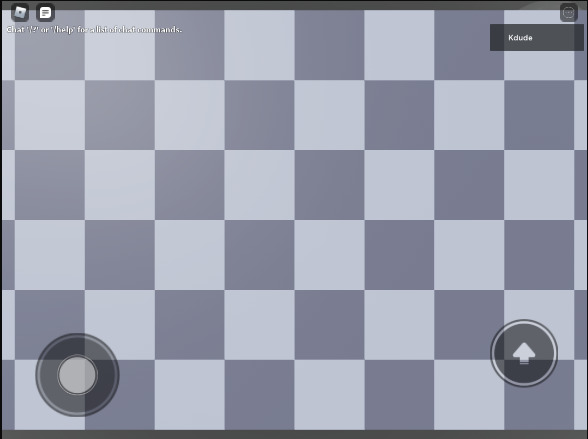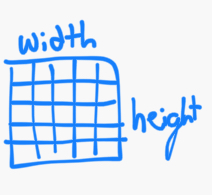# How would you ensure the camera can see every part when on different devices?

When setting the camera to a position, your vision can change depending on the aspect ratio of the screen you’re using.

On 1920x1080, you can see every single part 100%

But then on other resolutions, mostly box shaped ones, some parts can be only partially visible or not visible at allI can get the resolution from Camera.ViewportSize, and maybe I could increase the FOV or raise the camera position depending on certain aspect ratios, but maybe there’s a better solution out there.

1 Like

I’m assuming you’re gonna make a tile based game, so why don’t you just make the tiles disappear when they’re too far from the player? Also I don’t recommend you mess with FOV too much cause it starts to look bad.

The tiles were more of an example so you could tell that parts are off screen, but making parts disappear is the opposite of what I want, I’m trying to get every device to see all the parts, not some devices missing parts.

If I were you, I would move the camera farther away until all the parts fit. The only other solution I could think of is changing the shape of the subject, like making your parts fill a square.

You could prob use the diagonal field of view to calculate the proper distance

``````distanceAbove = 2 * math.tan(cam.DiagonalFieldOfView/2)/(math.sqrt(boxheight^2 + boxwidth^2))
``````

This should hopefully work
Youd prob wanna add a number onto the distance so theres a bit of a bounding box but whatever works

1 Like

I think this is a step in the right direction, but I’m confused about what the boxheight and boxwidth are, is that just the Y and X or Z of the entire grid? Doing that right now returns a very small negative number, so I think I got that wrong. Also, what would I do with the distanceAbove? Do I just add it to the Y of the camera position?

Oh sorry I made a few mistakes

``````distanceAbove = (math.sqrt(boxheight^2 + boxwidth^2))/(2 * math.tan(math.rad(cam.DiagonalFieldOfView/2)))
``````

This should hopefully give a good result

Also you would position the camera to be a distance of distanceAbove directly above the “box”, so maybe something like

``````Box.Position + Vector3.new(0,distanceAbove,0)
``````

Idk what youre doing though so it could be anything really

So I made a box exactly the size of all the parts, named PlayingField

And used

``````distanceAbove = (math.sqrt(workspace.PlayingField.Size.Y ^ 2 + workspace.PlayingField.Size.X ^ 2))/(2 * math.tan(math.rad(workspace.CurrentCamera.DiagonalFieldOfView / 2)))
``````

Not sure if it should be Y and X like I put, but I assumed boxheight meant Y, and boxwidth meant X.
And I get this:

I set the camera CFrame to the box’s position + distanceAbove, facing towards the box

``````workspace.CurrentCamera.CFrame = CFrame.new(workspace.PlayingField.Position + Vector3.new(0, distanceAbove, 0), workspace.PlayingField.Position)
``````

I’m assuming its that maybe I’m not supposed to use Y and X for height and width.

It should be the size of the box to the cameraThe ordering doesnt actually matter as long as its the correct values

Im not sure what the Y size refers to for you object, is it like this?

Ah ok so it should be X and Z. The size just refers to the size of the box.

So using X and Z, I get this. For some reason its rotated, I deleted one of the tiles to show you how the camera is positioned.

I guess this problem is a bit more complicated than I thought

The easiest way I can think of would be to find which size is bigger and use that as the “primary” side

``````local diagAngle = math.rad(Camera.DiagonalFieldOfView/2)
local distance
if width > height then
distance = width * math.tan(diagAngle)/math.cos(diagAngle)
else
distance = height/math.cos(diagAngle)
end
``````

This is a similar approach but it doesnt mistakenly assume that the ratio between the height and width is the exact same as the cameras

If you want to rotate it just swap height and width and rotate the cframe

Now we’re really far away.

Heres the code

``````local Camera = workspace.CurrentCamera

local width = workspace.PlayingField.Size.Z
local height = workspace.PlayingField.Size.X

local distanceAbove

if width > height then
distanceAbove = width * math.tan(diagAngle)/math.cos(diagAngle)
else
distanceAbove = height/math.cos(diagAngle)
end

workspace.CurrentCamera.CameraType = Enum.CameraType.Scriptable
workspace.CurrentCamera.CFrame = CFrame.new(workspace.PlayingField.Position + Vector3.new(0, distanceAbove, 0), workspace.PlayingField.Position)
``````

Sorry I got a bit confused, sorry for wasting your time trying a bunch of bad code

``````local Camera = workspace.CurrentCamera

local width = workspace.PlayingField.Size.Z
local height = workspace.PlayingField.Size.X

local distanceAbove
local diagAngle = math.atan(Camera.ViewportSize.Y/Camera.ViewportSize.X)

if width > height then
distanceAbove = width * math.tan(diagFov)/math.cos(diagAngle)
else
distanceAbove = height * math.tan(diagFov)/math.sin(diagAngle)
end

workspace.CurrentCamera.CameraType = Enum.CameraType.Scriptable
workspace.CurrentCamera.CFrame = CFrame.new(workspace.PlayingField.Position + Vector3.new(0, distanceAbove, 0), workspace.PlayingField.Position)
``````

Try this, I hope it works

Its all good man, not much on this topic and I’m bad at math so you’re helping out a lot.

We’re still pretty zoomed out. I switched the width and height and rotated the camera angle so its facing the right way now though.

Did you ever find out how to bring the camera in closer? I’m trying to replicate something like this in my game.

1 Like

Not yet, the script that was previously sent is a step in the right direction, but still off

I’ve actually figured out something that works when the fov is set to 90:

``````workspace.CurrentCamera.CFrame = CFrame.new(0, BoxSize * .5 )
``````

Tried a few things etc. but couldnt get it to work w anything other than 90 sadly.

1 Like

you could allow the player to orient there own camera?

1 Like